Courses

# Mirror Images Quiz I, Non Verbal Reasoning

## 25 Questions MCQ Test Non Verbal Reasoning | Mirror Images Quiz I, Non Verbal Reasoning

Description
This mock test of Mirror Images Quiz I, Non Verbal Reasoning for LR helps you for every LR entrance exam. This contains 25 Multiple Choice Questions for LR Mirror Images Quiz I, Non Verbal Reasoning (mcq) to study with solutions a complete question bank. The solved questions answers in this Mirror Images Quiz I, Non Verbal Reasoning quiz give you a good mix of easy questions and tough questions. LR students definitely take this Mirror Images Quiz I, Non Verbal Reasoning exercise for a better result in the exam. You can find other Mirror Images Quiz I, Non Verbal Reasoning extra questions, long questions & short questions for LR on EduRev as well by searching above.
QUESTION: 1

Solution:
QUESTION: 2

Solution:
QUESTION: 3

### Directions to Solve In each of the following questions you are given a combination of alphabets and/or numbers followed by four alternatives (1), (2), (3) and (4). Choose the alternative which is closely resembles the mirror image of the given combination. Question - Choose the alternative which is closely resembles the mirror image of the given combination.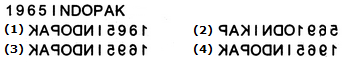Solution:
QUESTION: 4

In each of the following questions you are given a combination of alphabets and/or numbers followed by four alternatives (1), (2), (3) and (4). Choose the alternative which is closely resembles the mirror image of the given combination.

Question -

Choose the alternative which is closely resembles the mirror image of the given combination.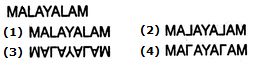Solution:
QUESTION: 5

5.Choose the alternative which is closely resembles the mirror image of the given combination.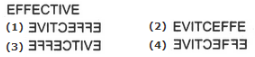Solution:
QUESTION: 6

In each of the following questions you are given a combination of alphabets and/or numbers followed by four alternatives (1), (2), (3) and (4). Choose the alternative which is closely resembles the mirror image of the given combination.

Question -

Choose the alternative which is closely resembles the mirror image of the given combination.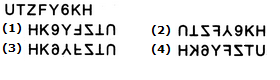Solution:
QUESTION: 7

In each of the following questions you are given a combination of alphabets and/or numbers followed by four alternatives (1), (2), (3) and (4). Choose the alternative which is closely resembles the mirror image of the given combination.

Question -

Choose the alternative which is closely resembles the mirror image of the given combination.Solution:
QUESTION: 8

Directions to Solve

In each of the following questions you are given a combination of alphabets and/or numbers followed by four alternatives (1), (2), (3) and (4). Choose the alternative which is closely resembles the mirror image of the given combination.

Question -

Choose the alternative which is closely resembles the mirror image of the given combination.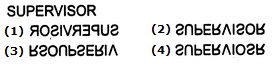Solution: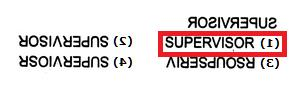QUESTION: 9

In each of the following questions you are given a combination of alphabets and/or numbers followed by four alternatives (1), (2), (3) and (4). Choose the alternative which is closely resembles the mirror image of the given combination.

Question -

Choose the alternative which is closely resembles the mirror image of the given combination.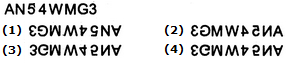Solution:
QUESTION: 10

In each of the following questions you are given a combination of alphabets and/or numbers followed by four alternatives (1), (2), (3) and (4). Choose the alternative which is closely resembles the mirror image of the given combination.

Question -

Choose the alternative which is closely resembles the mirror image of the given combination.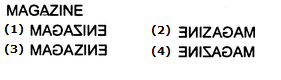Solution:
QUESTION: 11

In each of the following questions you are given a combination of alphabets and/or numbers followed by four alternatives (1), (2), (3) and (4). Choose the alternative which is closely resembles the mirror image of the given combination.

Question -

Choose the alternative which is closely resembles the mirror image of the given combination.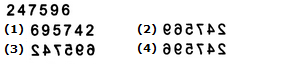Solution:
QUESTION: 12

In each of the following questions you are given a combination of alphabets and/or numbers followed by four alternatives (1), (2), (3) and (4). Choose the alternative which is closely resembles the mirror image of the given combination.

Question -

Choose the alternative which is closely resembles the mirror image of the given combination.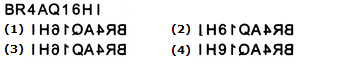Solution:
QUESTION: 13

In each of the following questions you are given a combination of alphabets and/or numbers followed by four alternatives (1), (2), (3) and (4). Choose the alternative which is closely resembles the mirror image of the given combination.

Question -

Choose the alternative which is closely resembles the mirror image of the given combination.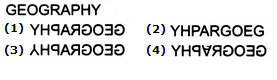Solution:
QUESTION: 14

In each of the following questions you are given a combination of alphabets and/or numbers followed by four alternatives (1), (2), (3) and (4). Choose the alternative which is closely resembles the mirror image of the given combination.

Question -

Choose the alternative which is closely resembles the mirror image of the given combination.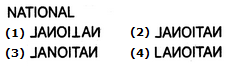Solution:
QUESTION: 15

In each of the following questions you are given a combination of alphabets and/or numbers followed by four alternatives (1), (2), (3) and (4). Choose the alternative which is closely resembles the mirror image of the given combination.

Question -

Choose the alternative which is closely resembles the mirror image of the given combination.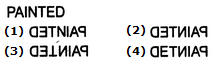Solution:
QUESTION: 16

In each of the following questions you are given a combination of alphabets and/or numbers followed by four alternatives (1), (2), (3) and (4). Choose the alternative which is closely resembles the mirror image of the given combination.

Question -

Choose the alternative which is closely resembles the mirror image of the given combination.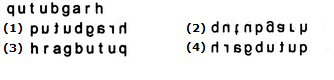Solution:
QUESTION: 17

In each of the following questions you are given a combination of alphabets and/or numbers followed by four alternatives (1), (2), (3) and (4). Choose the alternative which is closely resembles the mirror image of the given combination.

Question -

Choose the alternative which is closely resembles the mirror image of the given combination.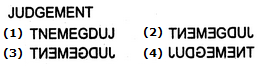Solution:
QUESTION: 18

In each of the following questions you are given a combination of alphabets and/or numbers followed by four alternatives (1), (2), (3) and (4). Choose the alternative which is closely resembles the mirror image of the given combination.

Question -

Choose the alternative which is closely resembles the mirror image of the given combination.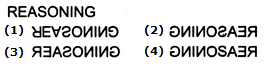Solution:
QUESTION: 19

In each of the following questions you are given a combination of alphabets and/or numbers followed by four alternatives (1), (2), (3) and (4). Choose the alternative which is closely resembles the mirror image of the given combination.

Question -

Choose the alternative which is closely resembles the mirror image of the given combination.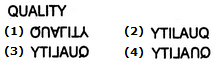Solution:
QUESTION: 20

Directions to Solve

In each of the following questions you are given a combination of alphabets and/or numbers followed by four alternatives (1), (2), (3) and (4). Choose the alternative which is closely resembles the mirror image of the given combination.

Question -

Choose the alternative which is closely resembles the mirror image of the given combination.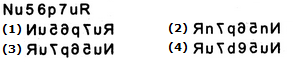Solution: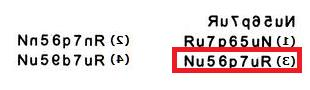QUESTION: 21

Directions to Solve

In each of the following questions you are given a combination of alphabets and/or numbers followed by four alternatives (1), (2), (3) and (4). Choose the alternative which is closely resembles the mirror image of the given combination.

Question -

Choose the alternative which is closely resembles the mirror image of the given combination.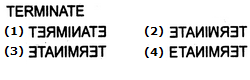Solution: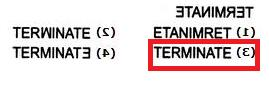QUESTION: 22

In each of the following questions you are given a combination of alphabets and/or numbers followed by four alternatives (1), (2), (3) and (4). Choose the alternative which is closely resembles the mirror image of the given combination.

Question -

Choose the alternative which is closely resembles the mirror image of the given combination.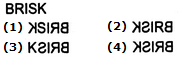Solution:
QUESTION: 23

Directions to Solve

In each of the following questions you are given a combination of alphabets and/or numbers followed by four alternatives (1), (2), (3) and (4). Choose the alternative which is closely resembles the mirror image of the given combination.

Question -

Choose the alternative which is closely resembles the mirror image of the given combination.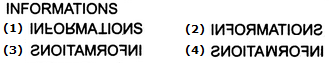Solution: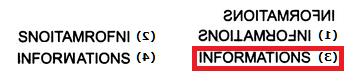QUESTION: 24

In each of the following questions you are given a combination of alphabets and/or numbers followed by four alternatives (1), (2), (3) and (4). Choose the alternative which is closely resembles the mirror image of the given combination.

Question -

Choose the alternative which is closely resembles the mirror image of the given combination.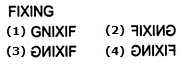Solution:
QUESTION: 25

In each of the following questions you are given a combination of alphabets and/or numbers followed by four alternatives (1), (2), (3) and (4). Choose the alternative which is closely resembles the mirror image of the given combination.

Question -

Choose the alternative which is closely resembles the mirror image of the given combination.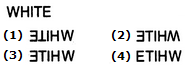Solution: